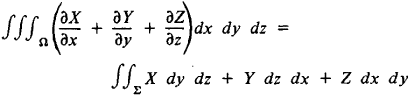The following article is from The Great Soviet Encyclopedia (1979). It might be outdated or ideologically biased.

a theorem relating an integral over a volume Ω bounded by a surface ∑ to an integral over the surface:where X, Y, and Z are functions of the point (x, y, z) belonging to the three-dimensional region Ω. Ostrogradskii’s theorem was developed by M. V. Ostrogradskii in 1828 and published in 1831. The theorem can be expressed in vector form as

∫∫∫Ω div pdr = ∫∫pn

where p is a vector field defined in Ω, is the element of volume, n is the exterior unit vector normal to the surface ∑, and is the element of surface.

In hydrodynamics Ostrogradskii’s theorem establishes the equivalence of two methods of computing the quantity of liquid flowing out of an envelope ∑ in a unit of time: (1) from the “output” of the point sources filling Ω (the left-hand side of the equality); and (2) from the velocities of the particles of liquid at the time the particles pass through the envelope ∑ (the right-hand side of the equality).

In 1824, Ostrogradskii extended the theorem to the case of an integral over an n-dimensional region. He published this result in 1838.